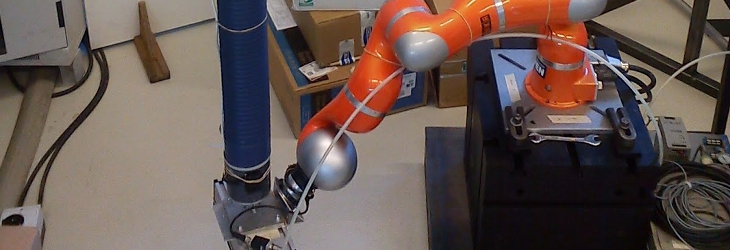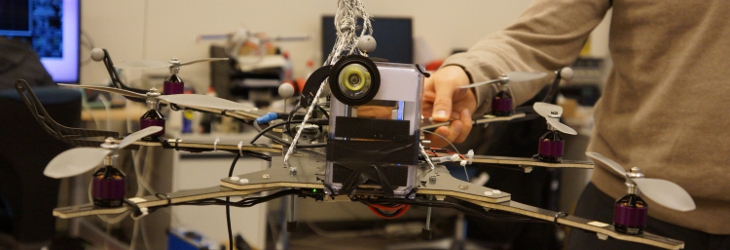# A message from the ECHORD coordinator Alois Knoll

## Public Summary Month 1/2012

During December 2011 and January 2012 the optimal control problem formulation was extended and an optimized motion was successfully transferred onto the KUKA robot arm. The demonstrator was changed to better match the workspace of the robot. Furthermore, there was an exchange of PhD students between Heidelberg University and LAAS-CNRS.

Previous problems with additional constraints such as ground collisions could be resolved which was due to an error in the formulation and the optimizations work now as expected. The code for the robot model was robustified by adding additional tests which will guarantee correctness also in the future.

Computed motions can now be exported and executed on the robot. So far the motions have to be checked manually to ensure that the robot does not collide with itself as self-collision is not yet implemented. Furthermore the motion has to be performed at a slower speed as the constraints posed in the optimal control formulation do not fully represent the safety parameters in the robot.

Previously we planned the demonstrator to be a track which moves around the robot in a non-linear path in the horizontal plane. However in this plane, the end-effector can only reach a limited dextrous workspace especially when obstacles are involved. We therefore changed it so that the path will be on a path that is aligned vertically. Furthermore, a smaller test-case scenario for the demonstrator was designed to test the motion generation pipeline. The work at LAAS-CNRS was dedicated to writing effecicient collision avoidance constraints, and starting to use the MUSCOD solver.

In order to implement fast distance constraints, we implement, using the Kineo Collision Detection (KCD) library, two new geometry types: segments and capsules. A segment is defined by its two end points, and a capsule is defined by its two axis end points and a radius. Collision detection and proximity query are also implemented for capsule-capsule, segment-segment, capsule-polyhedron, and segment-polyhedron tests.

The reason for which we use capsules and segments is the following: while the capsule type is very useful for fast collision detection, KCD does not allow the user to return a negative distance when two capsules are colliding. We hence define the segment geometry, retrieve the distance separating two segments (or a segment and a polyhedron). The user can then subtract the sum of capsule radii to obtain the real distance separating the equivalent capsules; if the distance is positive, then the capsules do not collide, otherwise they collide and the distance is equal to the penetration distance. This is an important feature for numerical optimization, as we can get a non-zero gradient even if the objects collide. We packaged all these classes in one library that can be used easily both by LAAS and IWR to define distance constraints.

Now that a capsule can be defined, a simplified model of the robot can be built by replacing all polyhedrons representing the bodies by their bounding capsules. The bounding capsule being a conservative approximation of a the real body geometry, this requires finding the minimum volume capsule that contains the set of all points of the polyhedron.

We proceed in two steps: first, the least-squares method is applied on the set of points to find the best line approximation. To make sure all points lie inside the capsule, the radius is set to the maximum distance between the line and the points, and the segment is defined as the minimum-length portion of line such that all points are insisde. The obtained capsule volume is not minimal, but it is a good approximation of the optimal solution.

Second, we take the computed capsule as initial guess and we solve the following numerical optimization problem: find the capsule paramters (end points and radius) that minimize the volume, such that the distance between the capsule and all points is negative, i.e. all points lie inside the capsule. We use the Ipopt solver that is available in RobOptim. We apply this two-stage optimization on all bodies of HRP-2. The obtained bounding capsules represent a fairly tight fit of the underlying geometries, especially convex volumes such as the arms and the legs.

Apart from that Antonio El Khoury from LAAS stayed at the IWR for two weeks. During the first week, he gave presentations about latest results in motion planning for anthropomorphic systems, and about the latest software implementations for the ECHORD project. The rest of the week was spent on following an introductory course to the MUSCOD solver, installing it and starting to use it on simple examples. During his stay, Antonio was able to successfully solve a simple optimization problem for HRP-2, using the dynamics model without any constraints.

Tags: